This handy Math in Focus Grade 1 Workbook Answer Key Chapter 3 Addition Facts to 10 detailed solutions for the textbook questions.

Math Journal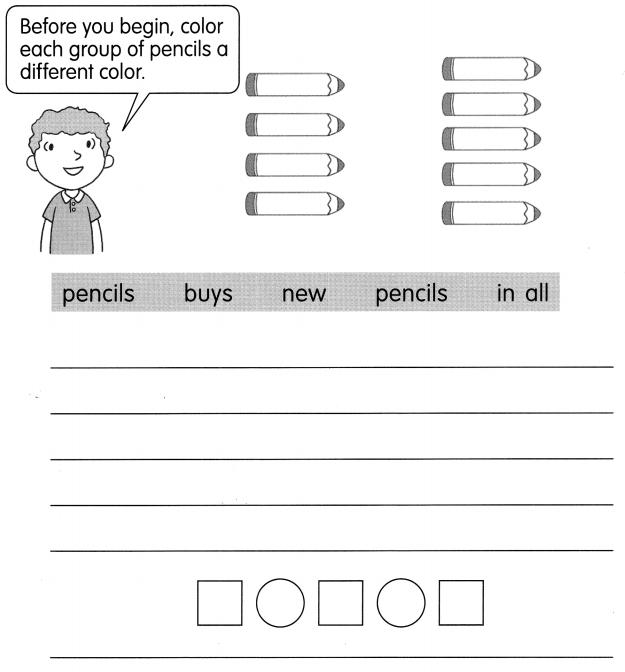Explanation: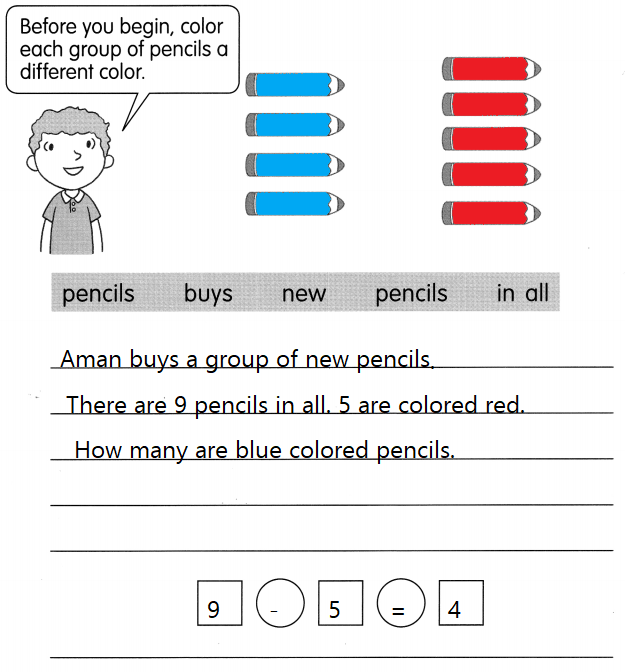Framed a question by using the given vocabulary
and formed an equation based on the story.

Challenging Practice

Solve.

Ivy and Reena have 10 prizes in all. They do not have the same number of prizes. How many prizes can Reena have?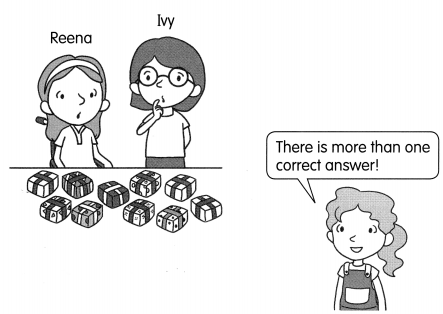Reena can have ___ prizes.

Problem Solving

Solve.
Ivy and Reena have 10 prizes in all. They do not have the same number of prizes.
so, 6 and 4 let us assume
6 + 4 = 10
Reena can have 6 prizes.

Lilian has these candles. Help her choose the correct number candle for her friend’s birthday.

• Cross out two numbers that add up to 5.
• Cross out two numbers that add up to 10.
• Look at the two numbers that are left.

Cross out the number that is the least.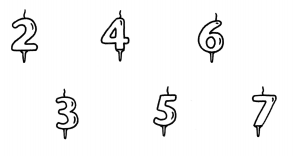The correct number candle is ____
The correct number candle is 7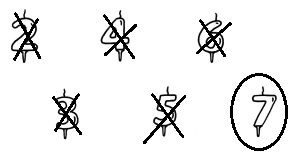Explanation:
2 + 3 = 5
so, crossed 2 and 3
6 + 4 = 10
so, crossed 6 and 4
remaining are 5 and 7
In that least is 5 so crossed that
remaining is 7

Chapter Review/Test

Vocabulary

Choose the correct word.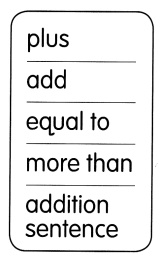Question 1.
You can ____ by counting on from the greater number.
Explanation:
You can add by counting on from the greater number.

Question 2.
2 + 3 = 5 is an ____
Explanation:
An addition sentence is a number sentence or simply an equation used to express addition.

Question 3.
3 plus 4 is ____ 7.
Equal to
Explanation:
3 + 4 = 7
The sum of 3 and 4 is 7

Question 4.
plus
Explanation:
The addition is represented with + symbol

Question 5.
6 is 2 ___ 4.
more than
Explanation:
6 is 2 more than 4.
4 + 2 = 6

Concepts and Skills

Add by counting on from the greater number.

Question 6.
3 + 6 = ___
9
Explanation:
The sum of 3a nd 6 is 9

Question 7.
7 + 1 = ____.
8
Explanation:
7 + 1 = 8
The sum of 7 and 1 is 8

Question 8.
2 + 8 = ___
10
Explanation:
2 + 8 = 10
The sum of 2 and 8 is 10

Question 9.
1 + 9 = ___
10
Explanation:
The sum of 1 and 9 is 10
1 + 9 = 10

Fill in the blanks.

Question 10.
____ is 3 more than 6.
9
Explanation:
9 is 3 more than 6.
6 + 3 = 9

Question 11.
____ is 2 more than 5.
7
Explanation:
7 is 2 more than 5.
5 + 2 = 7

Question 12.
___ is 4 more than 4.
8
Explanation:
8 is 4 more than 4.

Look at the pictures. Fill in the blanks.

Question 13.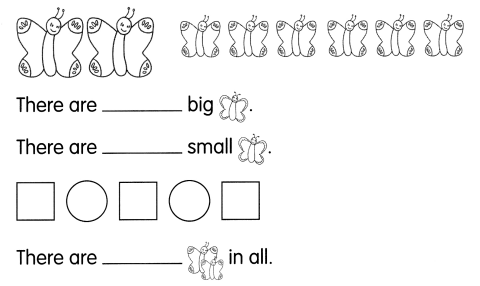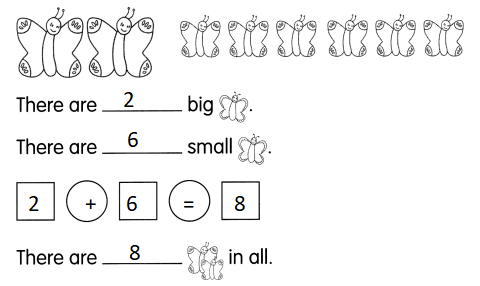Explanation:
An addition fact is the sum of two specific addends that will always equal the same result. 2 + 2 will always equal four. 7 + 8 will always equal 15.

Complete the number bonds. Fill in the blanks.

Question 14.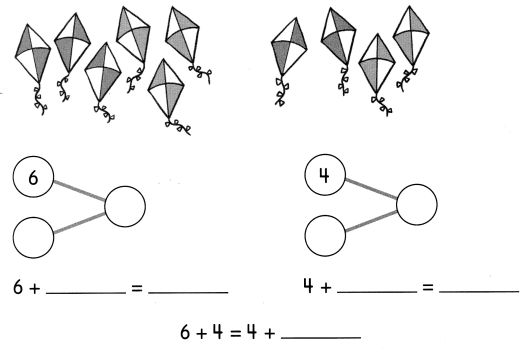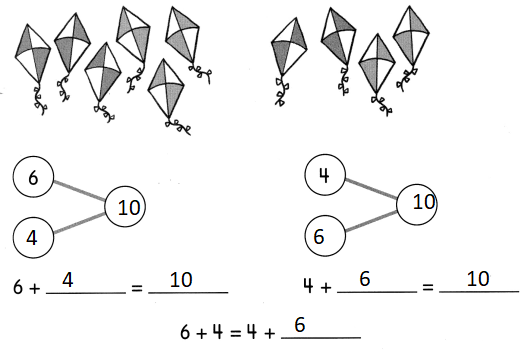Explanation:
An addition fact is the sum of two specific addends that will always equal the same result. 2 + 2 will always equal four. 7 + 8 will always equal 15.

Problem Solving

Solve.

Question 15.
Carlos has 3 brown belts. He has 2 black belts. How many belts does he have in all?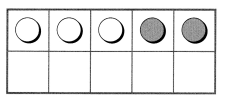3 + 2 = ___
Carlos has ___ belts in all.
3 + 2 = 5
Carlos has 5 belts in all.
Explanation:
Carlos has 3 brown belts. He has 2 black belts.
3 + 2 = 5
An addition fact is the sum of two specific addends that will always equal the same result. 2 + 2 will always equal four. 7 + 8 will always equal 15.

Question 16.
Jane has 4 bows. She gets 3 more bows. How many bows does she have now?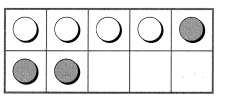4 + 3 = ___
Jane has __ bows now.
4 + 3 = 7
Jane has 7 bows now.
Explanation:
Jane has 4 bows. She gets 3 more bows.
4 + 3 = 7
An addition fact is the sum of two specific addends that will always equal the same result. 2 + 2 will always equal four. 7 + 8 will always equal 15.

Draw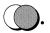Then Solve.

Question 17.
How many toys are there ¡n all?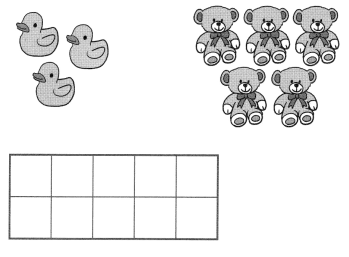3 + _____ = _____
There are ___ toys in all.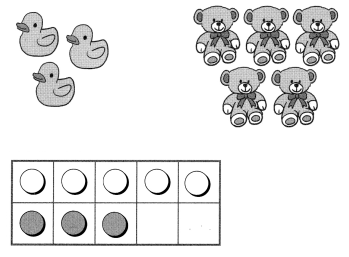Explanation:
5 + 3 = 8
There are 3 duck toys and 5 teddy toys
The are 8 toys in all

Look at the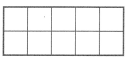in question 17 to answer the questions.

Question 18.
a.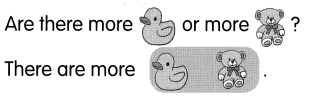Teddys
Explanation:
There are 3 duck toys and 5 teddy toys
5 > 3
so, teddy are more

b. How many more?
5 is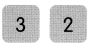more than 3.